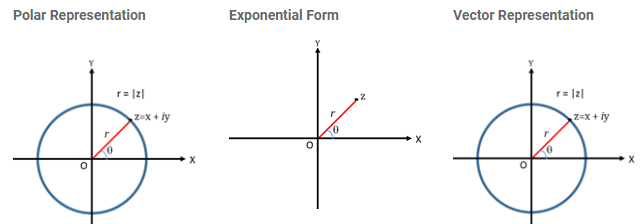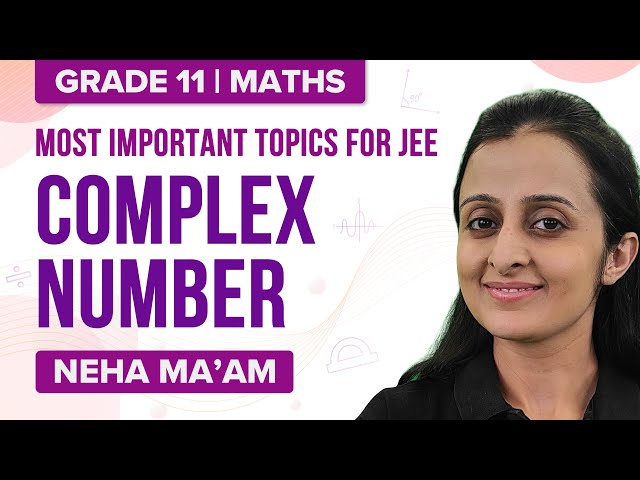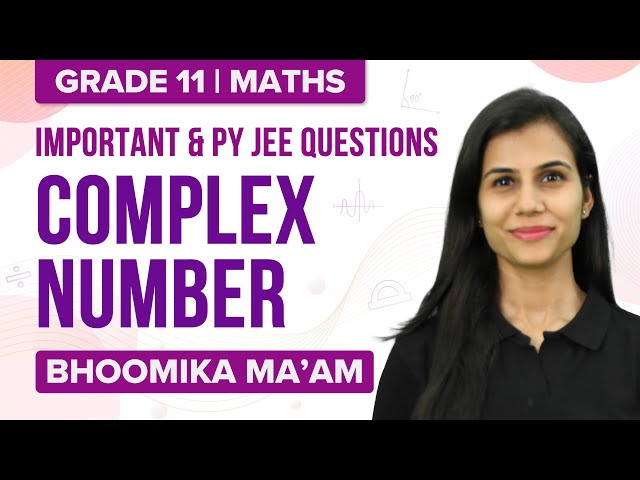Know more about the Repeaters Batch Know more about the Repeaters Batch

# Complex Numbers Solved Examples

Complex numbers are algebraic expressions which have real and imaginary parts. If the real part of a complex number is 0, then it is called “purely imaginary number”. This article gives insight into complex numbers definition and complex numbers solved examples for aspirants so that they can start with their preparation.

## Complex Number Definition

A number of the form z = x + iy where x is the real part, y is the imaginary part and x, y belong to a set of real numbers.

$$\begin{array}{l}\text{The value of i} = \sqrt{-1}.\end{array}$$
The value of i can’t be seen on the number line.

## Representation Of A Complex NumberPolar form of z : x = r cos θ, y = r sin θ

Exponential form of z : z = r e  (where e = cos θ + i sin θ)

## Modulus And Argument Of A Complex Number

If z = x + iy, then the modulus is denoted by

$$\begin{array}{l}|z|=\sqrt{a^2+b^2}.\end{array}$$
The angle made by the positive direction of the real axis can be defined as the argument of a complex number and denoted by arg(z).

The properties of modulus of complex numbers are as follows:

$$\begin{array}{l}1]\ |z_{1} z_{2}| = |z_{1}\cdot z_{2}|\\ 2]\ |z_{1} + z_{2}| \leq |z_{1} + z_{2}|\\ 3]\ |\frac{z_{1}}{z_{2}}|=\frac{|z_{1}|}{|z_{2}|}\\ 4]\ |z_{1} – z_{2}| \geq |z_{1} – z_{2}|\\\end{array}$$

The identities of arguments are as follows:

$$\begin{array}{l}1] \ \ {Arg}(z_{1}z_{2})\equiv \ {Arg}(z_{1})+\ {Arg}(z_{2}){\pmod {(-\pi ,\pi ]}},\\ 2] \ \ {Arg} {\biggl (}{\frac {z_{1}}{z_{2}}}{\biggr )}\equiv \ {Arg} (z_{1})-\ {Arg} (z_{2}){\pmod {(-\pi ,\pi ]}}.}\\ 3] \text{If} \ z \neq 0 \ and \ n \ is \ any \ integer, \ then \ {Arg}\left(z^{n}\right)\equiv n\ {Arg}(z){\pmod {(-\pi ,\pi ]}}.\end{array}$$

## Conjugate Of A Complex Number

A number consisting of equal real and imaginary part which is equal in magnitude but with opoosite sign can be termed as a complex comjugate of a complex number.

The properties of the conjugate of complex numbers are given by:

Let z and w be two complex numbers.

$$\begin{array}{l}1]\ {\overline {z+w}}={\overline {z}}+{\overline {w}}\\ 2]\ {\overline {z-w}}={\overline {z}}-{\overline {w}}\\ 3]\ {\overline {zw}}={\overline {z}}\;{\overline {w}}\\ 4]\ {\overline {\left({\frac {z}{w}}\right)}}={\frac {\overline {z}}{\overline {w}}},\quad {\text{if }}w\neq 0\\ 5]\ {\overline {z}}=z~\Leftrightarrow ~z\in \mathbb {R}\\ 6]\ {\overline {z^{n}}}=\left({\overline {z}}\right)^{n},\quad \forall n\in \mathbb {Z} \\ 7]\ \left|{\overline {z}}\right|=\left|z\right|\\ 8]\ {\left|z\right|}^{2}=z{\overline {z}}={\overline {z}}z\\ 9]\ {\overline {\overline {z}}}=z\\ 10] \ z^{-1}={\frac {\overline {z}}{{\left|z\right|}^{2}}},\quad \forall z\neq 0\end{array}$$

## Algebra Of Complex Numbers

The different rules for operations on complex numbers as follows:

$$\begin{array}{l}1] \ z_{1}+z_{2}=(a+bi)+(c+di)=(a+c)+(b+d)i\,}\\ 2] \ z_{1}-z_{2}=(a+bi)-(c+di)=(a-c)+(b-d)i\,}\\ 3] \ z_{1}z_{2}=(a+bi)(c+di)=ac+adi+bci+bdi^{2}=(ac-bd)+(bc+ad)i\,}\\ 4] \ \frac{z_{1}}{z_{2}}=\ {\displaystyle{\frac {ac+bd}{c^{2}+d^{2}}}+{\frac {bc-ad}{c^{2}+d^{2}}}i}.\\\end{array}$$

## Equality Of Complex Numbers

Consider two complex numbers z= (a+bi) and z= (c + di). They are said to be equal if their real and imaginary parts are equal that is

$$\begin{array}{l}z_{1} = z_{2} \rightarrow Re(z_{1}) = Re(z_{2})\ \text{and}\ \ Im (z_{1}) = Im (z_{2}).\end{array}$$

### Related articles

Complex numbers

Representation of a complex number

### Complex Numbers – Top 12 Most Important and Expected JEE Questions## Complex Number Examples

Solved examples on complex numbers are given below.

Example 1: If z1, z2, z3 are the vertices of an equilateral triangle ABC such that |z− i| = |z− i| = |z− i|, then what is the value of |z+z+ z3|?

Solution:

|z− i| = |z− i| = |z− i|

Hence, z1, z2, and z3, lie on the circle whose center is i.

Also, the circumcenter coincides.

[z1 + z2 + z3] / 3 = i

⇒ |z1 + z2 + z3| = 3

Example 2: What is the value of λ if the curve y = (λ + 1)x2 + 2 intersects the curve y = λx + 3 at exactly one point.

Solution:

As (λ + 1)x2 + 2 = λx + 3 has only one solution, so D = 0

⇒ λ2 − 4(λ + 1)(−1) = 0

Or

Λ2 + 4λ + 4 = 0

Or (λ + 2)2 = 0

Therefore, λ = −2

Example 3: If k + ∣k + z2∣ = |z|2 ;(k ∈ R), what is the possible argument of z?

Solution:

|k + z2| = |z2| − k = |z2| + |k|

⇒ k, z2 and 0 + i0 are collinear

⇒ arg (z2)= arg (k)

⇒ 2 arg (z) = π

⇒ arg (z) = π/2

Example 4: Let a≠0 and p(x) be a polynomial of degree greater than 2. If p(x) leaves remainders a and ?a when divided respectively, by x + a and x – a, the remainder when p(x) is divided by x2 − a2 is?

Solution:

We are given that p(-a) = a and p(a) = -a

[When a polynomial f(x) is divided by x – a, remainder is f[a]],

Let the remainder, when p(x) is divided by x2 − a2, be Ax+B.

Then,

p(x) = Q(x)(x2 − a2) + Ax + B ….. (1)

Where Q(x) is the quotient.

Putting x = a and -a in (1), we get

p(a) = 0 + Aa + B

⇒ −a = Aa + B …. (2)

And p(−a) = 0 − aA + B

⇒ a = −aA + B …….(3)

Solving (2) and (3), we get

B = 0 and A = -1

Hence, the required remainder is -x.

Example 5: If the roots of the equation x2+2ax+b=0 are real and distinct and they differ by at most 2m, then at what interval does b lie?

Solution:

Let the roots be α, β.

∴α + β = −2a and αβ = b

Given, |α − β| ≤ 2m

or |α − β|2 ≤ (2m)2 or(α + β)2 − 4ab ≤ 4m2 or 4a2 − 4b ≤ 4m2

⇒ a2 − m2 ≤ b and discriminant D > 0 or 4a2 − 4b > 0

⇒ a2 − m2 ≤ b and b < a2.

Hence, b ∈ [a2−m2,a2).

Example 6: Find the conjugate of (2 – i)(1 + 2i)/(2 + 3i)(3 – 2i).

Solution:

We have (2-i)(1+2i)/(2+3i)(3-2i) = (2-i+4i+2)/ (6+9i-4i+6)

= (4+3i) / (12+5i)

Multiply numerator and denominator by (12-5i)

(4+3i)(12-5i) / (12+5i)(12-5i) = (48+36i-20i+15)/(144+25)

= (63+16i) / 169

Hence the conjugate is (63+16i) / 169

Example 7: Solve the equation x2+3x+9 = 0

Solution:

We have x2+3x+9 = 0

b2-4ac = 32-4×1×9 = 9-36 = -27 < 0

∴ x = (-3+√-27)/2 or  (-3-√-27)/2  ( using equation [-b±√(b2-4ac)]/2a)

= (-3+√27 i)/2 or  (-3-√27 i)/2

## Complex Numbers – Important Topics## Complex Numbers – Important QuestionsTest your Knowledge on Complex Numbers